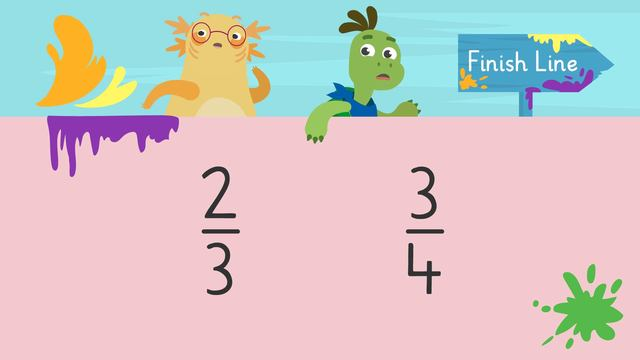# Comparing FractionsRating

Ø 5.0 / 1 ratings

The authorsTeam Digital
Comparing Fractions
CCSS.MATH.CONTENT.3.NF.A.3

## Basics on the topicComparing Fractions

### In This Video on Comparing Fractions 3rd Grade

Axel and Tank want to do a color run, but want to do the shorter distance! In order to identify which distance is shorter, they will be comparing fractions with different denominators. To find out more, watch this comparing fractions video 3rd grade! There is also a comparing fractions worksheet grade 3 at the end.

### 3rd Grade Comparing Fractions Example

What does comparing fractions mean? Fractions comparing means to take two fractions and work out if one is smaller or greater than the other fraction, or if they are both equal! When comparing two fractions, one of the strategies for comparing fractions you can use is comparing fractions with models, such as fraction bars.

When comparing fractions comparing:

• First, set up a fraction bar for the left side fraction. Since this fraction is one-half, use a one-half fraction bar.• Next, set up a fraction bar for the right side fraction. Since this fraction is one-half, use a one-half fraction bar.• Finally, compare the fraction bars! We can see the two fraction bars are equal, so use the equal to symbol.### Comparing Fractions Grade 3 - Summary

When comparing fractions third grade, remember:

• Set up a fraction bar for the left side fraction
• Set up a fraction bar for the right side fraction
• Compare the fraction bars and use the greater than, equal to, or less than symbol

This strategy can be used for comparing benchmark fractions. Below you will find a comparing fractions worksheet 3rd grade.

### TranscriptComparing Fractions

Axel and Tank are excited to sign up for a fun run! "We should do the shorter route!" "Okay, so we need to figure out which one is the shorter distance. Two thirds of a mile, or three fourths of a mile?" It looks like Axel and Tank will need help comparing fractions. When comparing fractions, we use greater than, less than, or equal to symbols. This means the value of the left side is greater than the value on the right side. This means the value of the left side is less than the value on the right side. This means the values on either side are equal. We can use fraction bars to model fractions and compare them. To compare fractions, first, set up a fraction bar for the fraction on the left side. Second, set up a fraction bar for the fraction on the right side. Then, compare the fraction bars. Determine if the fraction on the left is greater than, less than, or equal to the fraction on the right and label it using the correct symbol. Let's compare four-eights and one-fourth! First, set up a fraction bar for four-eights. The denominator, or bottom number, is eight, so divide the fraction bar into eight equal parts. The numerator, or top number, is four, so shade in four parts of the fraction bar. Now, set up a fraction bar for one-fourth. The denominator is four, so divide the fraction bar into four equal parts. The numerator is one, so shade in one part of the fraction bar. Finally, compare the fractions! We can see four-eights is bigger than one-fourth, so four-eights is greater than one-fourth! Now that we have practiced comparing fractions, let's help Axel and Tank solve which route is the shortest! The two distances were two thirds of a mile and three fourths of a mile! What is the first step? Create a fraction bar for two thirds. How many equal parts should it have? Three, because the denominator is three. How many parts should we shade in? Two, because the numerator is two. Now let's create a fraction bar for three fourths. How many equal parts are there? Four, because the denominator is four. How many parts should be shaded in? Three, because the numerator is three. What is the next step? Now we compare the fraction bars. Is two thirds greater than, less than, or equal to three fourths? Two thirds is less than three fourths, so Axel and Tank will run the two thirds of a mile route! Remember, to compare fractions, first, set up a fraction bar for the fraction on the left side. Second, set up a fraction bar for the fraction on the ride side. Next, compare the fraction bars. Determine if the fraction on the left side is greater than, less than, or equal to the fraction on the right side and label it using the correct symbol. "I didn't realize it was a color run Tank, did you?" "No Axel, But it was the best thing ever! Same again next year?" "If we must Tank, just for you."